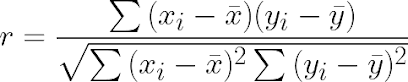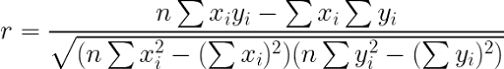# The Correlation Coefficient: What It Is and How It Can Help You in Machine Learning?

A Introduction to coefficient of correlation, what is it, How to calculate, and How it can be used in Machine Learning for improving Model accuracy.

## Introduction

How do you test the correlation between two variables? To answer this question, we first have to define what we mean by correlation. According to Merriam-Webster, correlation is defined as a relationship between two or more variables in which one variable changes whenever the other changes in some predictable way. A simple example of correlation would be if there were a relationship between an increase in temperature and an increase in ice cream sales during the summer months; we could predict that ice cream sales would rise when temperatures rose.

That's a simple example. When you’re working in machine learning, it’s useful to have an understanding of the correlation coefficient, or r. How do you calculate it? How can you interpret the number it generates? And how can you use this concept to improve your model accuracy? Find out all of that and more in this article on the correlation coefficient!

## What is the correlation coefficient? (Basic Definition)

The Coefficient of correlation is one of the popular statistical parameters that measures how closely two variables are related. In simple words, it indicates whether there exists a linear relationship between two variables or not. For example, if there is a positive relationship between height and weight then as height increases weight also increases. On the other hand, if there is no relation between two variables then we say that they are uncorrelated.

How can this measure be useful in machine learning? Well, the most useful case of correlation in machine learning is for predictive analysis. If there is a strong correlation between predicted values and actual values then we say that it has good accuracy. If we want to build a better predictive model, then we need to ensure that there exists some sort of relationship between predicted values and actual values.  The most commonly used method for calculating the correlation between two continuous variables is Pearson's correlation coefficient.

## The Pearson's correlation coefficient

The most popular way of finding the coefficient of correlation is by Pearson's correlation coefficient. This method is used when both variables are continuous, which means they can take any value from a range of values. In other words, they can be any real number between two given limits. For example, age can be any real number between 0 to 100 years old. If you want to calculate the correlation between age and height, you need to make sure that both variables are continuous before proceeding further with the calculation of the correlation coefficient.

Pearson's correlation is most commonly used in a regression analysis where it helps to understand how one variable changes when another variable change.  For example, if you want to know how your age affects your height, you can use Pearson's correlation coefficient. If you want to know how your weight affects your height, you need a different kind of correlation coefficient called Spearman's rank-order correlation coefficient (which we will discuss later).

The Pearson's correlation of coefficient 'r' is given by.Pearson's Correlation Formula

Where, r = Correlation Coefficient, xi = Value of x-variable, ẋ = Mean of x values, y = Values of y-variable, ȳ = Mean of y values.

When rearranging we'll get,Where n is the number of samples

## Calculating correlation coefficient

Consider the following x and y values:

Our goal is to find the correlation between these x and y values, so we can directly apply the values we figured from the table to the equation,

The correlation between x-variables and y-variables is 0.6863 which is approximately 68%. Note that the correlation is positive since the x and y variables are moving in the same direction in the graph.

## Positive correlation coefficient

A correlation is said to be positive if and only if a one-unit increase in X is associated with a one-unit increase in Y.

An example of a positively correlated pair of variables would be two variables measuring height and weight, whereas people become taller, they also tend to weigh more. This is because height correlates with weight; when one increases, so does the other. Another example of a positively correlated pair of variables would be stock prices and exchange rates. If stock prices rise, exchange rates are likely to do so as well. The reason for that is that people usually buy stocks with their local currency; therefore, when they want to buy stocks they will first have to convert their local currency into foreign currency (usually dollars), thus increasing demand for foreign currencies which will drive up their price relative to your local currency.

## Negative correlation coefficient

If a correlation coefficient is negative, it means that when one variable goes up, another variable tends to go down. For example, if you notice that bad weather causes your sales to dip on a day-to-day basis, it would be reasonable to assume there’s a negative correlation between rainy days and daily sales. It’s important to note that negative correlations are not necessarily causal; just because two variables tend to move together doesn’t mean one causes changes in another. That said, many popular machine learning algorithms are able to detect whether positive or negative correlations exist between input variables so they can adjust their predictions accordingly. This process of adjusting prediction is known as feature scaling.

## Graphical Interpretation of Correlation

How can we represent the correlation coefficient graphically? We can use a scatter plot for this purpose. If they are perfectly correlated then the plot will be a straight line with a slope of 1. If there is no correlation, then there will be no pattern visible in the data, so it should appear as random points scattered around an imaginary line with zero slopes (the x-axis). The closer our points are to being on a straight line, or zero slope means that there is a stronger correlation between these two variables.

The correlation graph of the x and y values in the above case will look like this:Correlation Coefficient Graph(Seaborn)

## Applications of correlation coefficients

How correlations can be applied in the real world? Let’s say you have a dataset of exam scores from your students. If you want to predict their scores for upcoming exams, you might find that there is a strong correlation between their performance on past exams and their performance on future ones. This makes sense; if someone does well on an exam, they are likely to do well on another one covering similar material. In cases like these, you could use a correlation coefficient to quantify how much of one variable (past performance) predicts another (future performance). The coefficient of correlation quantifies how strongly two variables are related.

Some applications of correlation include:

• 1. Predicting future performance based on past performance (as described above)
• 2. Estimating how much of one variable can be explained by another (e.g., how much of a person’s height is explained by their weight?)
• 3. Quantifying how well a model fits a dataset
• 4. Comparing two datasets to see if they have similar features or not
• 5. Evaluating whether two variables are independent or dependent on each other